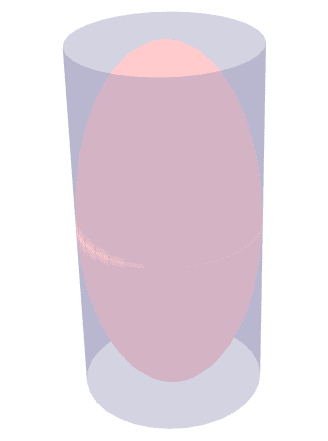# Area of a cylinder enclosing an ellipsoid

• I
I.m not absolutely sure if this comes under physics or maths, so apologies if I've put it in the wrong place.

It is well known that if a sphere is exactly enclosed by a cylinder, the area of the curved surface of the cylinder is equal to hat of the sphere.

Does this also apply if the cylinder encloses an ellipsoid, ie the surface created when an ellipse is rotated around one of its axes? Or does it only hold for spheres?

vanhees71
Gold Member
I don't understand the geometrical setup you have in mind. Do you have a picture?

Sorry I don't, but the case of a sphere would be when the cylinder exactly fitted around it. The ellipsoid would wither be a long oval shape or else a heavily flattened sphere - again with the cylinder exactly fitting round it.

Hope that helps

vanhees71
Gold Member
Ok, for the sphere then you have a cylinder with radius ##R## of the sphere and height ##2R##. It's surface is ##2 \pi R^2+2 \pi R 2R=6 \pi R^2## (the areas of the two circles of radius ##R## and the mantle) this is not the surface of the sphere, which is ##4 \pi R^2##.

Ok, for the sphere then you have a cylinder with radius ##R## of the sphere and height ##2R##. It's surface is ##2 \pi R^2+2 \pi R 2R=6 \pi R^2## (the areas of the two circles of radius ##R## and the mantle) this is not the surface of the sphere, which is ##4 \pi R^2##.

But the area of the curved surface of the cylinder does equal that of the sphere.

Ibix
2020 Award
You could just look up the equation for the surface area of an ellipsoid of revolution with axes ##a## and ##b## and see if it's equal to ##4\pi ab##...

A.T.
I.m not absolutely sure if this comes under physics or maths, so apologies if I've put it in the wrong place.
Definitively maths.

It is well known that if a sphere is exactly enclosed by a cylinder, the area of the curved surface of the cylinder is equal to hat of the sphere.

Does this also apply if the cylinder encloses an ellipsoid, ie the surface created when an ellipse is rotated around one of its axes? Or does it only hold for spheres?
To answer such questions quickly you should consider the extreme cases: What happens to the two surfaces if you flatten the sphere completely?

Baluncore
"This shows that a very elongated ellipsoid has an area of p2ab (e is close to 1 and b is much less than a), which is about 21.46% less than the lateral area of the circumscribed cylinder (4pab), whereas these two areas are equal in the case of a sphere, as noted by Archimedes of Syracuse (c.287-212BC)."

•Meir Achuz and Delta2
mfb
Mentor
It turns out there is no nice closed formula for the area of an ellipsoid. That means it's not only different from the cylinder area: In addition there is no other nice shape that has the same area.

•Delta2
Many thanks to everyone who has replied.

Initially I thought the answer might be yes, h inasmuas a sphere is only the limiting case for an ellipsoid with all axes equal. However, having heard these replies (and found several formulae online) I can see it ain't.

Cheers.

vanhees71
Gold Member
I still don't get the picture for the elliptic case. Do you mean a cylinder then with an elliptic cross section, which is the curve common to the cylinder and the ellipsoid? Then your question seems to be a pretty tough problem.

Delta2
Homework Helper
Gold Member
I still don't get the picture for the elliptic case. Do you mean a cylinder then with an elliptic cross section, which is the curve common to the cylinder and the ellipsoid? Then your question seems to be a pretty tough problem.
No i dont think he means a cylinder with elliptic cross section. He means a prolate or oblate ellipsoid instead. A generic ellipsoid has three axis a,b,c. In the prolate or oblate ellipsoid two of a,b,c are equal. In a sphere all 3 are equal.

•Ibix
Ibix•